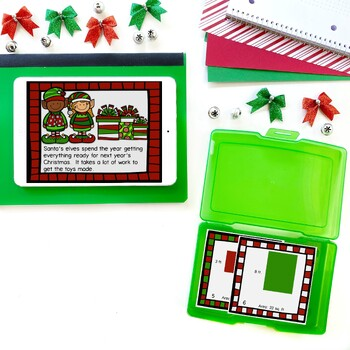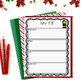# An Area & Perimeter Christmas Unit: Elving AroundSubject
Resource Type
File Type
Zip (12 MB|124 pages)
Standards
\$6.00
• Product Description
• Standards

Looking for an alternative to the area & perimeter robot? This elf themed integrated writing and math unit is perfect for teaching area and perimeter in December! Complete with a craft, writing activity, printables, and task cards, these Christmas area and perimeter activities will be a hit in your classroom!

WHAT'S INCLUDED:
4 projectable e-books for direct instruction
• Elf craftivity with writing project
• 2 sets of task cards
• 20 pages of printables (5 sets of 4 pages)

This interactive unit features a projectable e-book that introduces students to area and perimeter through the elves' work at the North Pole. Students practice determining both the area and the perimeter through printables and task cards, and by measuring their own elf's torso. There is an elf craftivity that can be used to build paragraphing skills as students write a biography of their elf. Quadrilaterals are provided with dimensions in cm, in, ft, and yd form.

The unit also includes additional e-books, task cards, and printables practicing additional area and perimeter standards. There are 3 additional projectable e-books for direct instruction on finding missing side lengths using area and perimeter, recognizing area as additive and finding area of rectilinear figures.

SKILLS INCLUDED:
• Measuring area with unit squares
• Finding area by tiling
• Finding area by multiplying side lengths
• Recognizing area as additive; finding the area of rectilinear figures
• Finding perimeter given side lengths
•Finding perimeter of polygons with unknown side lengths
• Finding unknown side lengths using perimeter
• Finding unknown side lengths unsing area
• Finding area using perimeter; finding perimeter using area

OTHER AREA & PERIMETER PRODUCTS:
Area & Perimeter Story Problems Task Cards

CLICK HERE to follow me and be notified of future products as soon as they are posted.

_______________________________________________________________

You may not redistribute, edit, sell, or otherwise post this product on the internet. You may, however, post a link for others to purchase themselves.

Recognize area as additive. Find areas of rectilinear figures by decomposing them into non-overlapping rectangles and adding the areas of the non-overlapping parts, applying this technique to solve real world problems.
Use tiling to show in a concrete case that the area of a rectangle with whole-number side lengths 𝘢 and 𝘣 + 𝘤 is the sum of 𝘢 × 𝘣 and 𝘢 × 𝘤. Use area models to represent the distributive property in mathematical reasoning.
Solve real world and mathematical problems involving perimeters of polygons, including finding the perimeter given the side lengths, finding an unknown side length, and exhibiting rectangles with the same perimeter and different areas or with the same area and different perimeters.
Multiply side lengths to find areas of rectangles with whole-number side lengths in the context of solving real world and mathematical problems, and represent whole-number products as rectangular areas in mathematical reasoning.
Find the area of a rectangle with whole-number side lengths by tiling it, and show that the area is the same as would be found by multiplying the side lengths.
Total Pages
124 pages